paper 里提出的方法主要分为两大模块：VAM(visual-aware ranking model) 和 HBM(hybrid bandit model), 总体的模块图如下所示，VAM 即上面提到的基于 list-wise 优选做 exploitation 模块，HBM 则是基于 badnit model 做 exploration 模块，下面也主要从这两个模块进行介绍。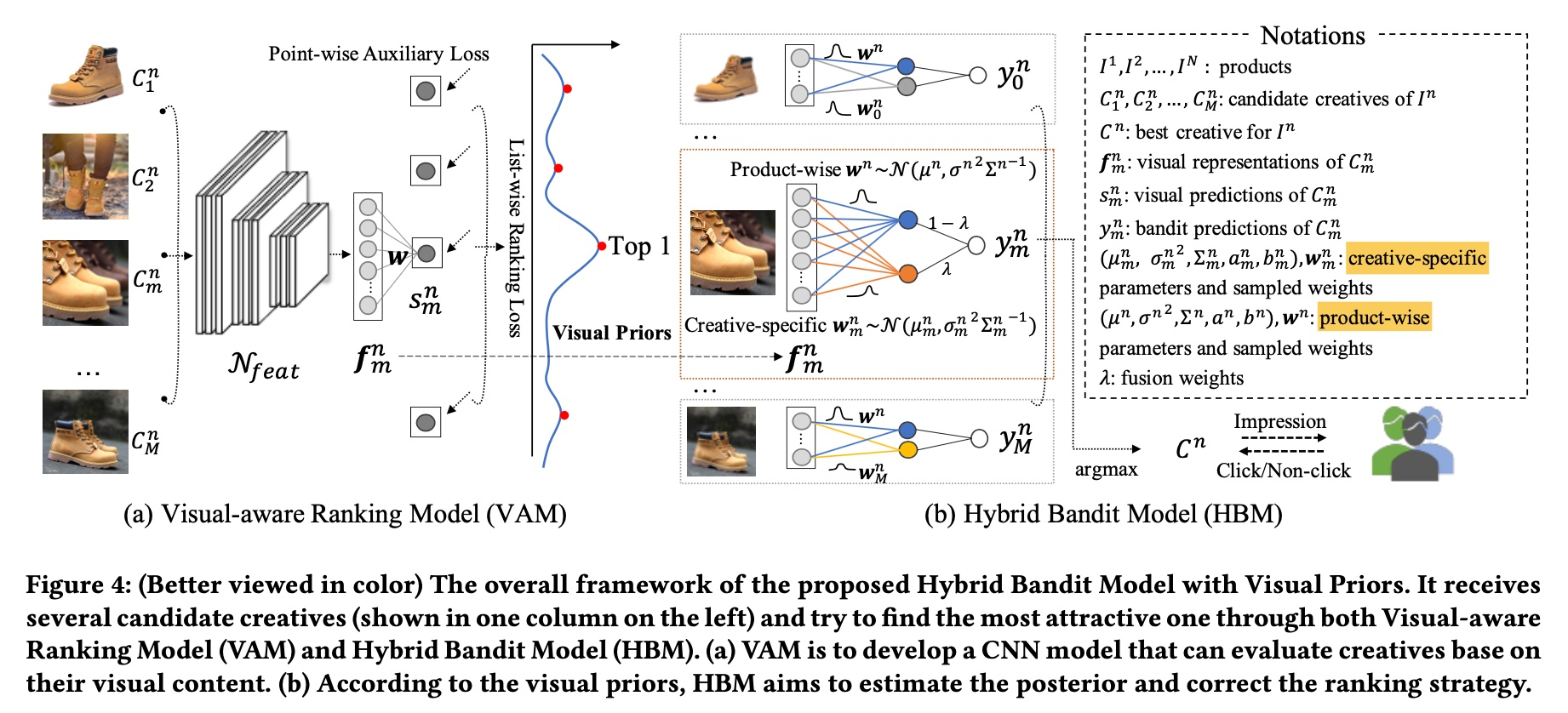## VAM

### list-wise loss

list-wise 是 learning to rank 里一种建模方式，另外两种分别是 point-wise 和 pair-wise，常见的 ctr/cvr 预估都是采用 point-wise 的方式；

VAM 采用的 list-wise loss 即是第二篇 paper 中的提出的 loss，其流程如下图所示，更详细的推导可参考上面第二篇 paper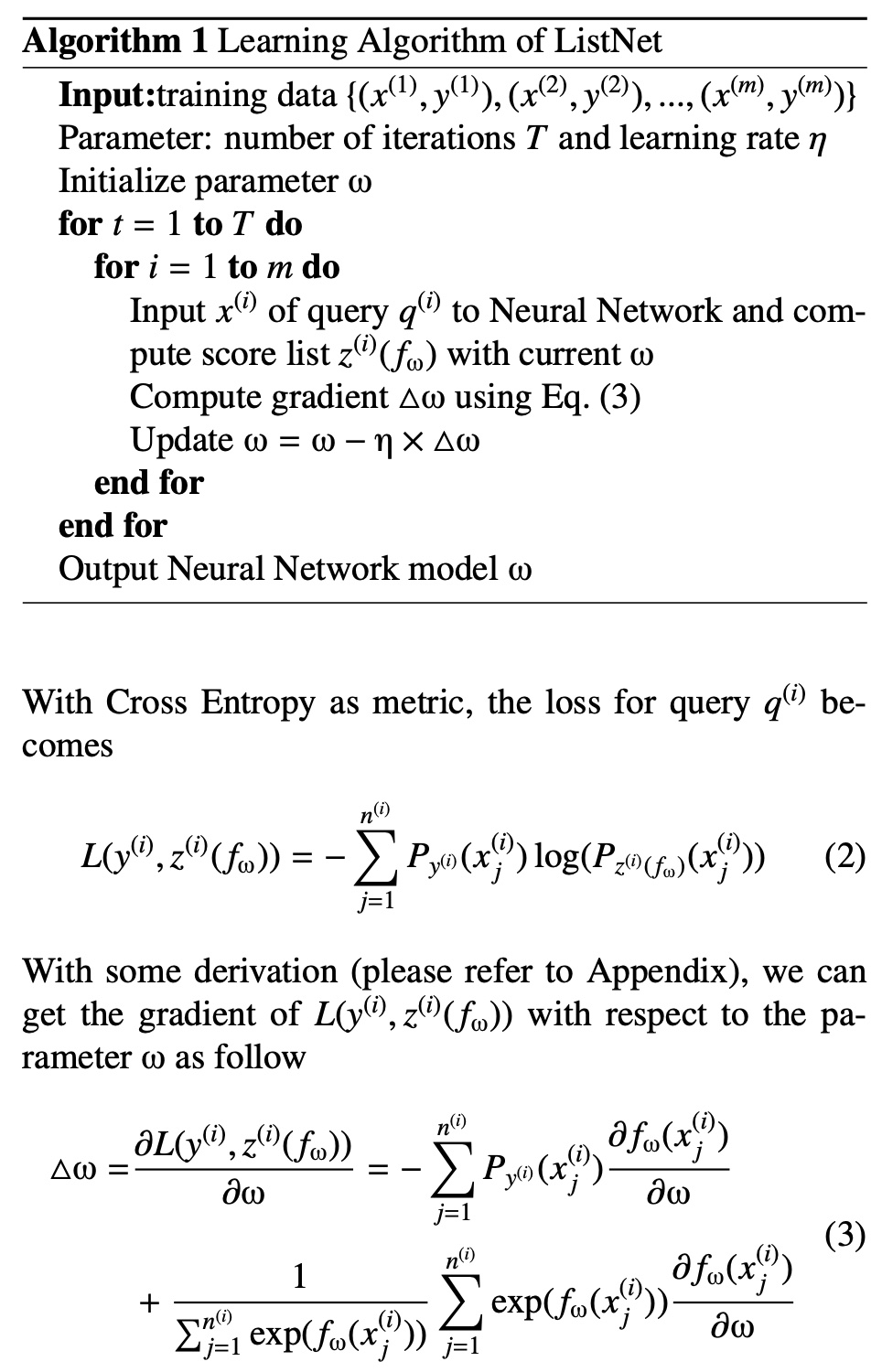### VAM loss

$$p_{m}^{n} = \frac{\exp(s_m^n)}{\sum_{i=1}^{M}\exp(s_i^n)}$$

$$y_{rank}(C_m^n) = \frac{\exp(CTR(C_m^n), T) }{\sum_{i=1}^{M}\exp(\exp(CTR(C_i^n), T)}$$

$y_{rannk}(C_m^n)$ 中的 $T$ 的作用是 adjust the scale of the value so that make the probability of top1 sample close to 1，则对于第 $n$ 个 product, 其 list-wise loss 如下所示

$$L_{rank}^{n}=-\sum_{m}y_{rank}(C_m^n)\log(p_{m}^{n})$$

$$L_{reg}^{n}=\sum_{m} ||CTR(C_m^n) - s_m^n||_{2}$$

$$L^{n} = L_{rank}^{n} + \gamma L_{reg}^{n}$$

### noise mitigation

，其思想是基于贝叶斯学派给点击数 clicks 和 CTR 值整个先验分布，这样遇到极端值时也有分布约束，导致最终的值不会太离谱，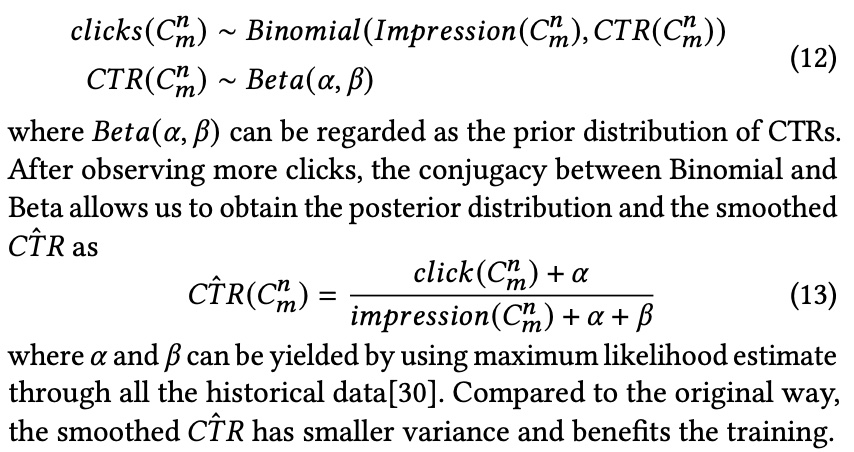## HBM

HBM 本质上是一个 Bayesian Linear Regression，从名字大概就能猜测，这个是贝叶斯学派的方法，即认为模型参数是服从一个分布，通过从分布里采样达到 exploration 的目的，其推导过程如下

$$y = f^T\widetilde{w} + \epsilon$$

paper 里将 $\epsilon$ 先验分布假设为一个正态分布即 $\epsilon \thicksim N(0, \sigma^2)$, 同样将 $\widetilde{w}|\sigma^2$ 假设为一个正态分布，两者互为共轭

$$\sigma^2 \thicksim IG(a, b)$$

$$\widetilde{w}|\sigma^2 \thicksim N(\mu, \epsilon^2 \Sigma^{-1})$$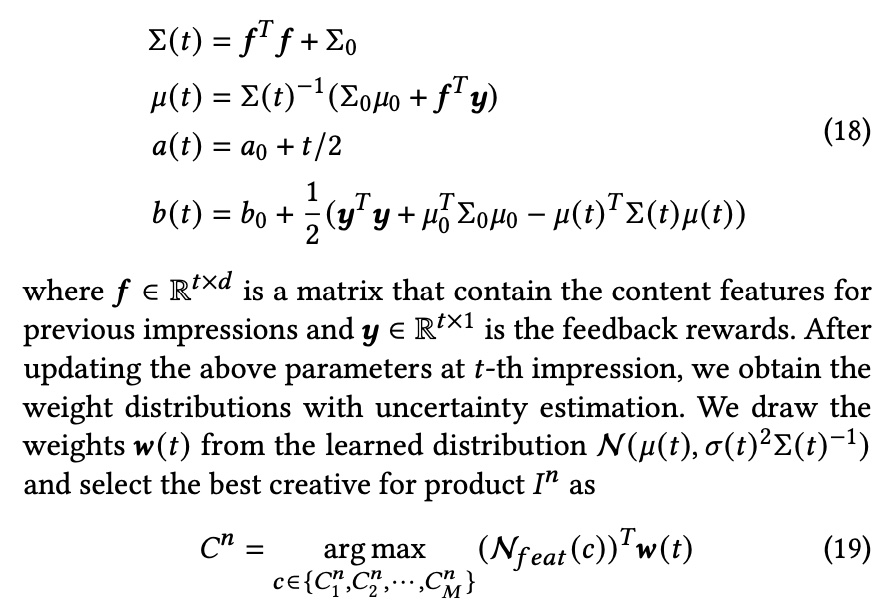$$y_m^n = {f_m^n}^{T}w^n + {f_m^n}^{T}w_m^n$$

paper 这样做的原因是

This simple linear assumption works well for small datasets, but becomes inferior when dealing with industrial data. For example, bright and vivid colors will be more attractive for women’s top while concise colors are more proper for 3C digital accessories. In addition to this product-wise characteristic, a cre- ative may contain a unique designed attribute that is not expressed by the shared weights. Hence, it is helpful to have weights that have both shared and non-shared components.

$$\lambda = (1+e^{\frac{-impression(I^n)+\theta_2}{\theta_1}})^{-1}$$

$$y_m^n = (1-\lambda){f_m^n}^{T}w^n + \lambda{f_m^n}^{T}w_m^n$$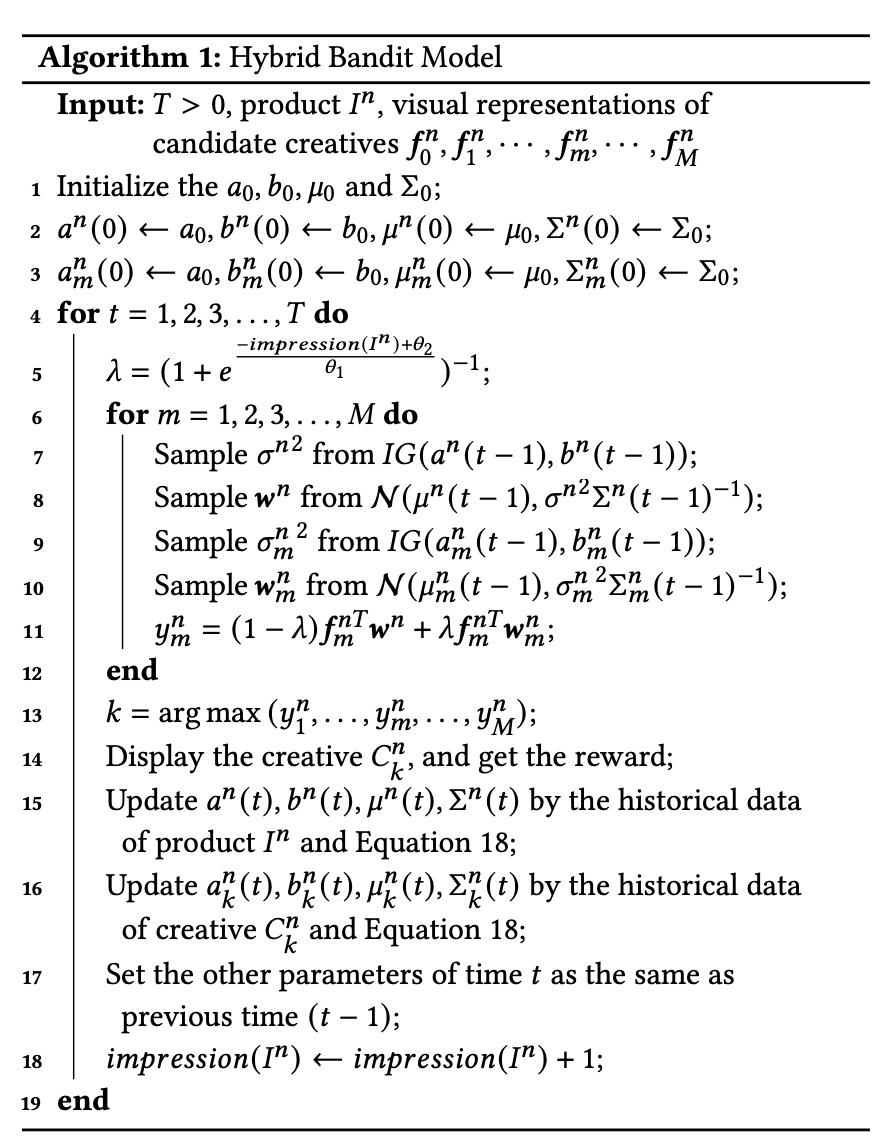## 实验

paper 里采用了 2 个评估指标：Simulated CTR(sCTR) 和 Cumulative regret, 前者模拟 online learning 过程，后者则是评估 bandit model ，两者计算方法如下，但是好像这两个指标不是非常通用？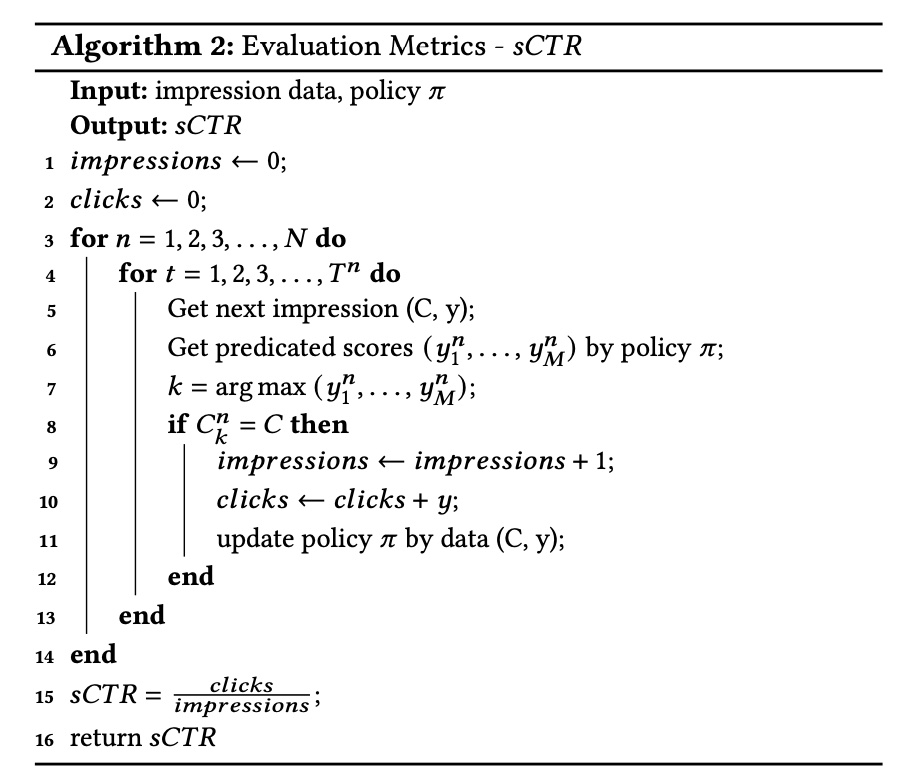## 小结

(1) VAM 利用投后数据(ctr), 通过 list-wise 方法学习出 creative 的 visual representation
(2) HBM 利用 VAM 的 visual representation 通过 bandit model，来实现 exploration 部分，同时考虑了 product-wise 和 creative-wise 建模和预估 score 的融合

(1) product-wise 和 creative-wise 的分数时，$\lambda$参数只考虑到 product-wise 的信息，没能很好体现 creative-wise的权重，具体原因上面说了
(2) 广告系统通常是召回+精排的环节，精排往往是 creative 粒度的，上面提出的系统未必能完整地融入现有的广告系统，倒是 VAM 训练得到的 visual representation 作为精排模型的一个 feature 是一种可能的方法
(3) VAM 已经可以对候选创意做优选了，为什么还需要 HBM 来做 exploration？或者说 exploration 是为了拿什么收益? 根据笔者的经验，在广告系统中 exploration 意味着破坏原来系统 feedback loop 所形成的分布，这往往会破坏系统由于马太效应形成的稳态，往往会造成收入的下降，相对应兑换的是一些生态指标或者信仰指标的提升。